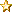###Author Topic: How to do power of calculations  (Read 224 times)

#### Nick_W

• Full Member
•• Posts: 215##### How to do power of calculations
« on: July 27, 2016, 10:26:30 am »
Hi,

I'm trying to calculate a "power of" value using floats.

The calculation involves pressure (variables are Floats) and has this in it: (atmospheric / seaLevel) ^ 0.1903

The error is "Operand(0) is not a byte, short, or Integer in bit-wise Xor (^)"

Now, the bitwise operator is ^ but the power of operator is also ^, and I want the power-of operator. How does VB.Net type languages differentiate?

In C I use pow(A, B) where I want A^B (to the power of, not bit-wise).

What's the equivalent?

Thanks,

#### mc-Abe##### Re: How to do power of calculations
« Reply #1 on: July 27, 2016, 04:38:16 pm »
The ^ operator is a indeed the XOR operator. You can use this for power operation: (atmospheric / seaLevel).Pow(0.1903)

If you look in section 16.6 of the manual, you can see all the float functions.

#### Nick_W

• Full Member
•• Posts: 215##### Re: How to do power of calculations
« Reply #2 on: August 19, 2016, 09:38:14 am »
Thanks,

There seems to be something wrong with the Pow function. Here is my code:

Code: [Select]
`    Public Function readAltitude(seaLevel As Float) As Float                // Equation taken from BMP180 datasheet (page 16):                // Note that using the equation from wikipedia can give bad results        // at high altitude.                Dim atmospheric As Float = readPressure()        Dim factor As Float = atmospheric / seaLevel        A_factor = factor.Pow(0.1903) // value used for debuging        Return 44330.0 * (1.0 - (factor.Pow(0.1903)))    End Function`
This gives incorrect values. Checking, I find the factor is correct - for atmospheric at 1000.802795 and sealLevel at 1000.00 the factor is 1.000802795 (correct). If I then raise this to the power of 0.1903 (which is 1/5.255) I get 0.189175 which is not correct - this should be 1.00015 (aprox).

Any idea what is going on here?

Thanks.

#### mc-John

• Global Moderator
• Full Member
•• Posts: 212##### Re: How to do power of calculations
« Reply #3 on: August 19, 2016, 10:47:12 am »
This is a bug. The argument are reserved. So 2 to power of 10 gives 100 instead of 1024.

We will solve this ASAP but there is a workaround to solve this. See code below:

Code: [Select]
`        Dim factor As Float = 1.000802795        Dim res As Float        res = factor.Pow(0.1903) ' res = 0.1900467        res = (0.1903).Pow(factor) ' res = 1.000153`
Hope this helps

-John-

#### Nick_W

• Full Member
•• Posts: 215##### Re: How to do power of calculations
« Reply #4 on: August 19, 2016, 12:53:07 pm »
OK, thanks,

The workaround seems to work.